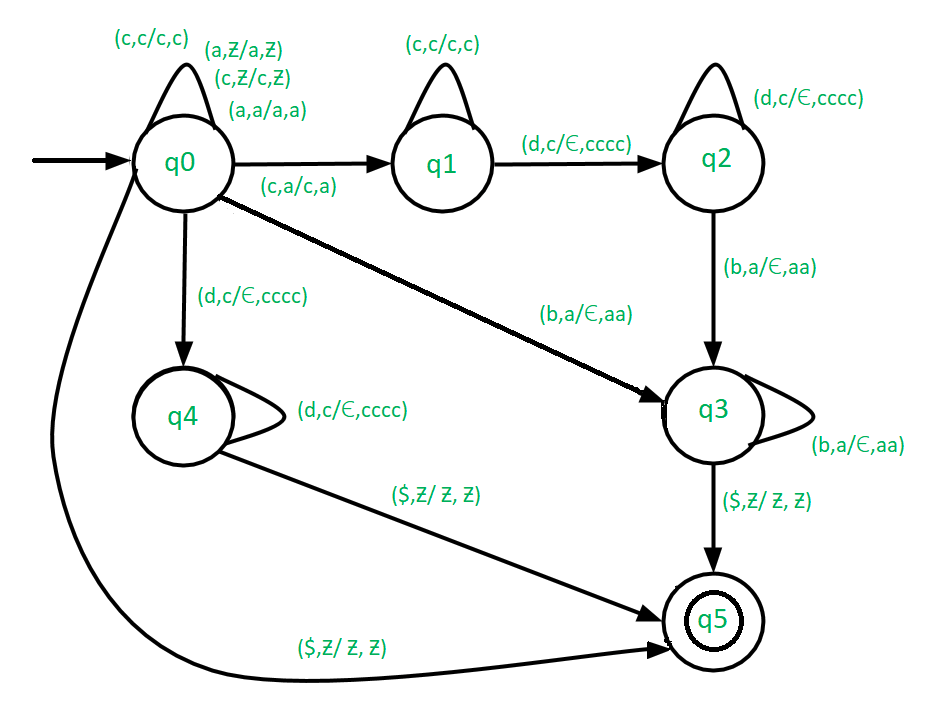# Construct Pushdown automata for L = {a(2*m)c(4*n)dnbm | m,n ≥ 0}

• Difficulty Level : Hard
• Last Updated : 17 Aug, 2018

Prerequisite – Pushdown automata, Pushdown automata acceptance by final state
PDA plays a very important role in task of compiler designing. That is why there is a need to have a good practice on PDA. Our objective is to construct a PDA for L = {a(2*m)c(4*n)dnbm | m,n ≥ 0}

Examples –

```Input: aaccccdb
Output: Accepted

Input: aaaaccccccccddbb
Output: Accepted

Input: acccddb
Output: Not Accepted ```

Approach used in this PDA –
There can be four cases while processing the given input string.

Case-1: m=0 – In this cases the input string will be of the form {c(4*n)dn}. In this condition, keep on pushing c’s in the stack until we encounter with ‘d’. On receiving ‘d’ check if top of stack is ‘c’, then pop ‘cccc’ from the stack. Keep on popping cccc’s until all the d’s of the string are processed. If we reach to the end of input string and stack becomes empty, then reached to the final state, i.e., accepts the input string else move to dead state.

Case-2: n=0 – In this cases the input string will be of the form {a(2*m)bm}. In this condition, keep on pushing a’s in the stack until we encounter with ‘b’. On receiving b check if top of stack is ‘a’, then pop ‘aa’ from the stack. Keep on popping aa’s until all the b’s of the string are processed. If we reach to the end of input string and stack becomes empty, then reached to the final state i.e., accepts the input string else move to dead state.

Case-3: m, n>0 – In this cases the input string will be of the form {(a(2*m)c(4*n)dnbm}. In this condition, keep on pushing a’s and c’s in the stack until we encounter with ‘d’. On receiving d check if top of stack is ‘c’, then pop ‘cccc’ from the stack. Keep on poping cccc’s until all the d’s of the input string are processed. Then On receiving b check if top of stack is ‘a’, then pop ‘aa’ from the stack. Keep on poping aa’s until all the b’s of the input string are processed. If we reach to the end of input string and stack becomes empty, then reach to final state i.e., accept the input string else move to dead state.

Case-4: m, n=0 – In this case the input string will be empty. Therefore directly jump to final state.Note –

1. We use (b, a/€, aa) to pop 2 a's, i.e., aa when encounter with b.
2. We use (d, c/€, cccc) to pop 4 c's, i.e., cccc when encounter with d.
My Personal Notes arrow_drop_up